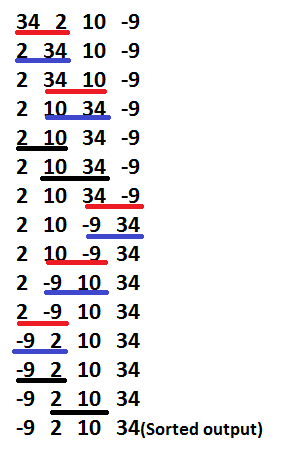Related Articles

# Gnome Sort

• Difficulty Level : Easy
• Last Updated : 31 Mar, 2021

Gnome Sort also called Stupid sort is based on the concept of a Garden Gnome sorting his flower pots. A garden gnome sorts the flower pots by the following method-

• He looks at the flower pot next to him and the previous one; if they are in the right order he steps one pot forward, otherwise he swaps them and steps one pot backwards.
• If there is no previous pot (he is at the starting of the pot line), he steps forwards; if there is no pot next to him (he is at the end of the pot line), he is done.

Input –

```Array- arr[]
Total elements - n```

Algorithm Steps

1. If you are at the start of the array then go to the right element (from arr to arr).
2. If the current array element is larger or equal to the previous array element then go one step right

```                   if (arr[i] >= arr[i-1])
i++;```

1. If the current array element is smaller than the previous array element then swap these two elements and go one step backwards

```                       if (arr[i] < arr[i-1])
{
swap(arr[i], arr[i-1]);
i--;
}```

1. Repeat steps 2) and 3) till ‘i’ reaches the end of the array (i.e- ‘n-1’)
2. If the end of the array is reached then stop and the array is sorted.

Example-

`   34 2 10 -9`

• Underlined elements are the pair under consideration.
• “Red” colored are the pair which needs to be swapped.
• Result of the swapping is colored as “blue”Below is the implementation of the algorithm.

## C++

 `// A C++ Program to implement Gnome Sort``#include ``using` `namespace` `std;` `// A function to sort the algorithm using gnome sort``void` `gnomeSort(``int` `arr[], ``int` `n)``{``    ``int` `index = 0;` `    ``while` `(index < n) {``        ``if` `(index == 0)``            ``index++;``        ``if` `(arr[index] >= arr[index - 1])``            ``index++;``        ``else` `{``            ``swap(arr[index], arr[index - 1]);``            ``index--;``        ``}``    ``}``    ``return``;``}` `// A utility function ot print an array of size n``void` `printArray(``int` `arr[], ``int` `n)``{``    ``cout << ``"Sorted sequence after Gnome sort: "``;``    ``for` `(``int` `i = 0; i < n; i++)``        ``cout << arr[i] << ``" "``;``    ``cout << ``"\n"``;``}` `// Driver program to test above functions.``int` `main()``{``    ``int` `arr[] = { 34, 2, 10, -9 };``    ``int` `n = ``sizeof``(arr) / ``sizeof``(arr);` `    ``gnomeSort(arr, n);``    ``printArray(arr, n);` `    ``return` `(0);``}`

## Java

 `// Java Program to implement Gnome Sort` `import` `java.util.Arrays;``public` `class` `GFG {``    ``static` `void` `gnomeSort(``int` `arr[], ``int` `n)``    ``{``        ``int` `index = ``0``;` `        ``while` `(index < n) {``            ``if` `(index == ``0``)``                ``index++;``            ``if` `(arr[index] >= arr[index - ``1``])``                ``index++;``            ``else` `{``                ``int` `temp = ``0``;``                ``temp = arr[index];``                ``arr[index] = arr[index - ``1``];``                ``arr[index - ``1``] = temp;``                ``index--;``            ``}``        ``}``        ``return``;``    ``}` `    ``// Driver program to test above functions.``    ``public` `static` `void` `main(String[] args)``    ``{``        ``int` `arr[] = { ``34``, ``2``, ``10``, -``9` `};` `        ``gnomeSort(arr, arr.length);` `        ``System.out.print(``"Sorted sequence after applying Gnome sort: "``);``        ``System.out.println(Arrays.toString(arr));``    ``}``}` `// Code Contributed by Mohit Gupta_OMG`

## Python

 `# Python program to implement Gnome Sort` `# A function to sort the given list using Gnome sort``def` `gnomeSort( arr, n):``    ``index ``=` `0``    ``while` `index < n:``        ``if` `index ``=``=` `0``:``            ``index ``=` `index ``+` `1``        ``if` `arr[index] >``=` `arr[index ``-` `1``]:``            ``index ``=` `index ``+` `1``        ``else``:``            ``arr[index], arr[index``-``1``] ``=` `arr[index``-``1``], arr[index]``            ``index ``=` `index ``-` `1` `    ``return` `arr` `# Driver Code``arr ``=` `[ ``34``, ``2``, ``10``, ``-``9``]``n ``=` `len``(arr)` `arr ``=` `gnomeSort(arr, n)``print` `"Sorted seqquence after applying Gnome Sort :"``,``for` `i ``in` `arr:``    ``print` `i,` `# Contributed By Harshit Agrawal`

## C#

 `// C# Program to implement Gnome Sort``using` `System;` `class` `GFG {``    ` `    ``static` `void` `gnomeSort(``int``[] arr, ``int` `n)``    ``{``        ``int` `index = 0;` `        ``while` `(index < n)``        ``{``            ``if` `(index == 0)``                ``index++;``            ``if` `(arr[index] >= arr[index - 1])``                ``index++;``            ``else` `{``                ``int` `temp = 0;``                ``temp = arr[index];``                ``arr[index] = arr[index - 1];``                ``arr[index - 1] = temp;``                ``index--;``            ``}``        ``}``        ``return``;``    ``}` `    ``// Driver program to test above functions.``    ``public` `static` `void` `Main()``    ``{``        ``int``[] arr = { 34, 2, 10, -9 };``        ` `        ``// Function calling``        ``gnomeSort(arr, arr.Length);` `        ``Console.Write(``"Sorted sequence after applying Gnome sort: "``);` `        ``for` `(``int` `i = 0; i < arr.Length; i++)``            ``Console.Write(arr[i] + ``" "``);``    ``}``}` `// This code is contributed by Sam007`

## PHP

 `= ``\$arr``[``\$index` `- 1])``            ``\$index``++;``        ``else``        ``{``            ``\$temp` `= 0;``            ``\$temp` `= ``\$arr``[``\$index``];``            ``\$arr``[``\$index``] = ``\$arr``[``\$index` `- 1];``            ``\$arr``[``\$index` `- 1] = ``\$temp``;``            ``\$index``--;``        ``}``    ``}``    ``echo` `"Sorted sequence "``,``         ``"after Gnome sort: "``;``    ``for` `(``\$i` `= 0; ``\$i` `< ``\$n``; ``\$i``++)``        ``echo` `\$arr``[``\$i``] . ``" "``;``    ``echo` `"\n"``;``}` `// Driver Code``\$arr` `= ``array``(34, 2, 10, -9);``\$n` `= ``count``(``\$arr``);` `gnomeSort(``\$arr``, ``\$n``);` `// This code is contributed``// by Sam007``?>`

## Javascript

 ``

Output:

`Sorted sequence after applying Gnome sort: -9 2 10 34`

Time Complexity – As there are no nested loop (only one while) it may seem that this is a linear O(N) time algorithm. But the time complexity is O(N^2). This is because the variable – ‘index’ in our program doesn’t always gets incremented, it gets decremented too.
However this sorting algorithm is adaptive and performs better if the array is already/partially sorted.
Auxiliary Space –  This is an in-place algorithm. So O(1) auxiliary space is needed.
This article is contributed by Rachit Belwariar. If you like GeeksforGeeks and would like to contribute, you can also write an article and mail your article to contribute@geeksforgeeks.org. See your article appearing on the GeeksforGeeks main page and help other Geeks.